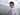# 15. 三数之和

December 13, 2019

https://leetcode-cn.com/problems/3sum/

``````func threeSum(nums []int) [][]int {
var res [][]int
if len(nums) < 3 {
return res
}
set := make(map[int]int)
flag := make(map[int]map[int]bool)
for _, num := range nums {
set[num]++
flag[num] = make(map[int]bool)
}
for num1 := range set {
set[num1]--
for num2, count2 := range set {
if count2 == 0 || flag[num1][num2] {
continue
}
set[num2]--
num3 := 0 - num1 - num2
count3 := set[num3]
if count3 > 0 {
res = append(res, []int{num1, num2, num3})
flag[num1][num2] = true
flag[num1][num3] = true
flag[num2][num1] = true
flag[num2][num3] = true
flag[num3][num1] = true
flag[num3][num2] = true
}
set[num2]++
}
set[num1]++
}

return res
}``````